mixtures and alligation practice problems with solutions

mixtures and alligation practice problems with solutions
5 (100%) 2 vote[s]
Question 1: A mixture of 24 litres contains water and milk in the ratio 3: 5. How much water must be added to this mixture to reverse the ratio?
[A] 20 litres
[B] 24 litres
[C] 18 litres
[D] 16 litres
Option # 4

Explanation

Solution :

let X litre of water be added, initial quantity of water 3/8  x 24  =  9.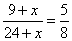X=16 litres.

Question 2: A trader mixes two varieties of tea costing Rs 40 per kg and rupees 50 per kg in the ratio 5: 4. If the mixture is sold at rupees 48 per kg question, then what is the gain percentage?
[A] 7.5 %
[B] 8%
[C] 2.5 %
[D] 5%
Option # 2

Explanation

Solution :

let the quantities of two varieties of tea be "5X" kg and "4X" kg respectively.

Thus, cost price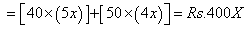..… (1)

and sales price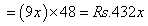…..  (2)

Profit=(2)-(1)=Rs. 32x

profit %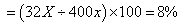Question 3: Two containers of equal volume contains milk and water in the ratio 3: 5 and 5: 3, respectively. If the contents of both containers are emptied into a third one, what would be the ratio of milk to water in that container?
[A] 1: 1
[B] 9: 25
[C] 25: 9
[D] None of these
Option # 1

Explanation

Solution :

Let the volume of both the container b x units each. Thus, the first container would contain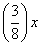units of milk and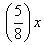units of water, whereas the second container would contain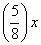units of milk andunits of water. When the content of both are empty into a third one it would have,

Milk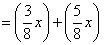…(1)

And water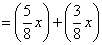….(2)

Thus, the required  ratio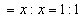.

Question 4: From two ornament Weighing 18 gram and 24 gram containing gold and silver in the ratio of 2: 1 and 5: 1 respectively, a new ornament is made. What is the amount of gold in the new ornament?
[A] 10 gram
[B] 12 gram
[C] 32 gram
[D] 28 gram
Option # 3

Explanation

Solution :

from the first ornament, gold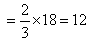From the second ornament gold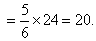Thus, total gold=32 gm.

Question 5: A and B are two alloys of gold and copper prepared by mixing metals in proportions 5: 3 and 5: 11 respectively. If equal quantities of this alloys are melted to a third alloy C, what would be the proportion of gold and copper in the alloys thus formed?
[A] 25: 33
[B] 33: 25
[C] 15: 17
[D] 17: 15
Option # 3

Explanation

Solution :

required proportion of gold and silver is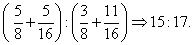Practie Problems Sets